(机器学习必知必会)

# 一、概率和统计的区别

Lary Wasserman 在 All of Statistics 的序言里有说过概率论和统计推断的区别：

The basic problem that we study in probability is:
Given a data generating process, what are the properities of the outcomes?

The basic problem of statistical inference is the inverse of probability:
Given the outcomes, what can we say about the process that generated the data?

• 概率是已知模型和参数，推数据（例如均值，方差，协方差等）
• 统计是已知数据，推模型和参数（例如高斯分布、指数分布等）

# 二、概率函数和似然函数的区别 $P(x|\theta)$

• 如果$\theta$是已经确定的，$x$是变量，那这个函数就叫做概率函数（probability function），它描述已知模型的情况下，不同的样本点x出现的概率是多少。
• 如果$x$是已经确定的，$\theta$是变量，那这个函数就叫做似然函数（likelihood function），它描述对于不同的模型参数$\theta$，出现$x$这个样本点的概率是多少。

# 四、贝叶斯学派：最大后验估计 $\underset{\theta}{\operatorname{argmax}} P(x \mid \theta) P(\theta)$

## 抛硬币实例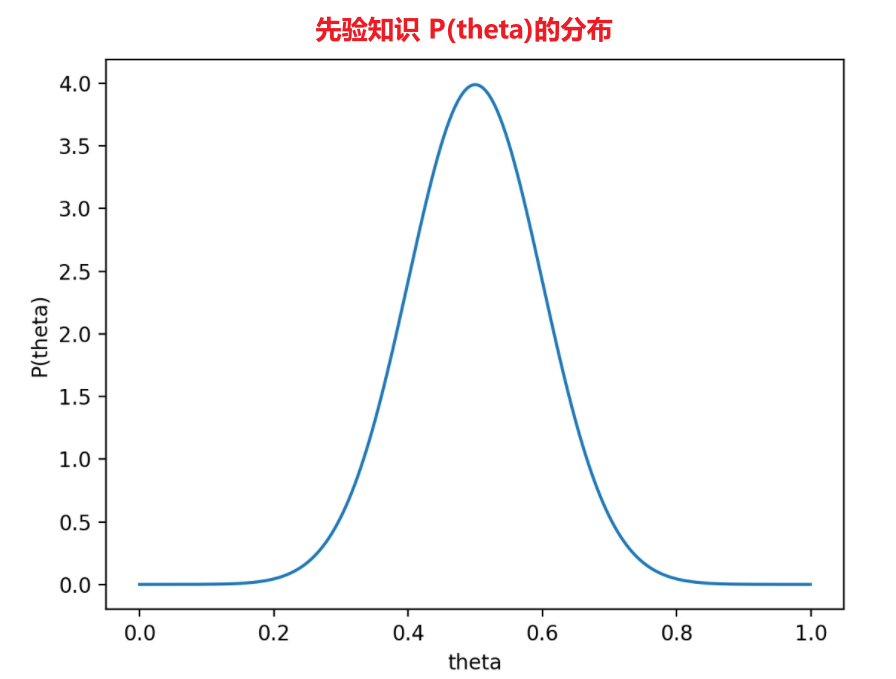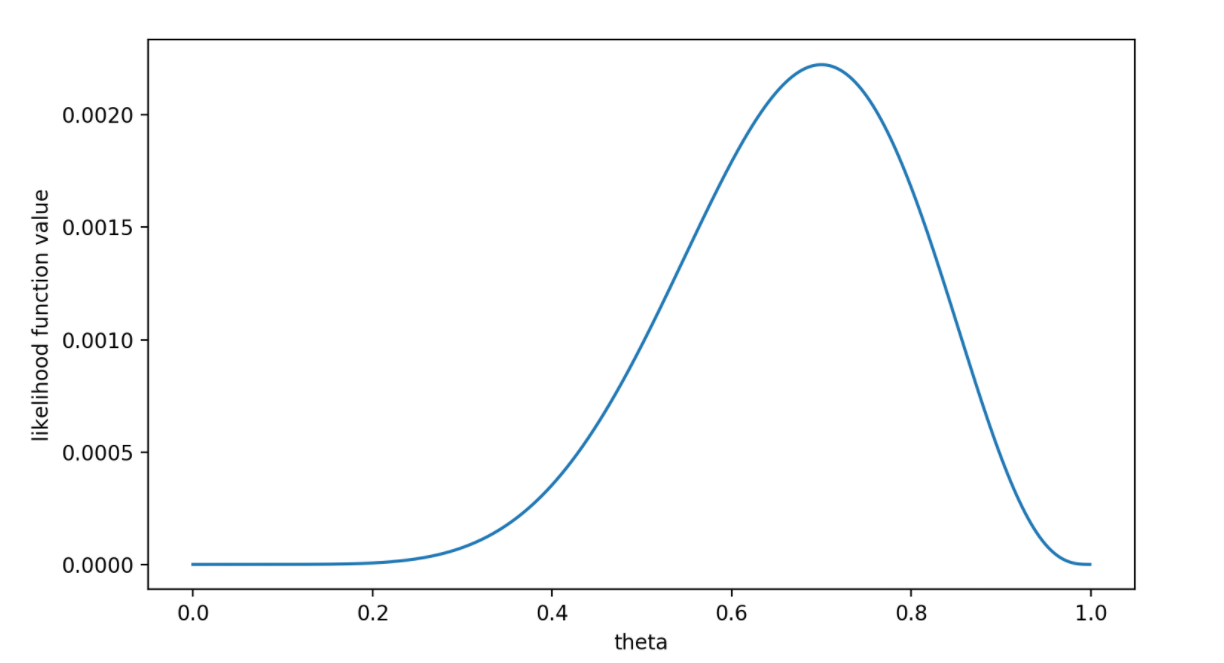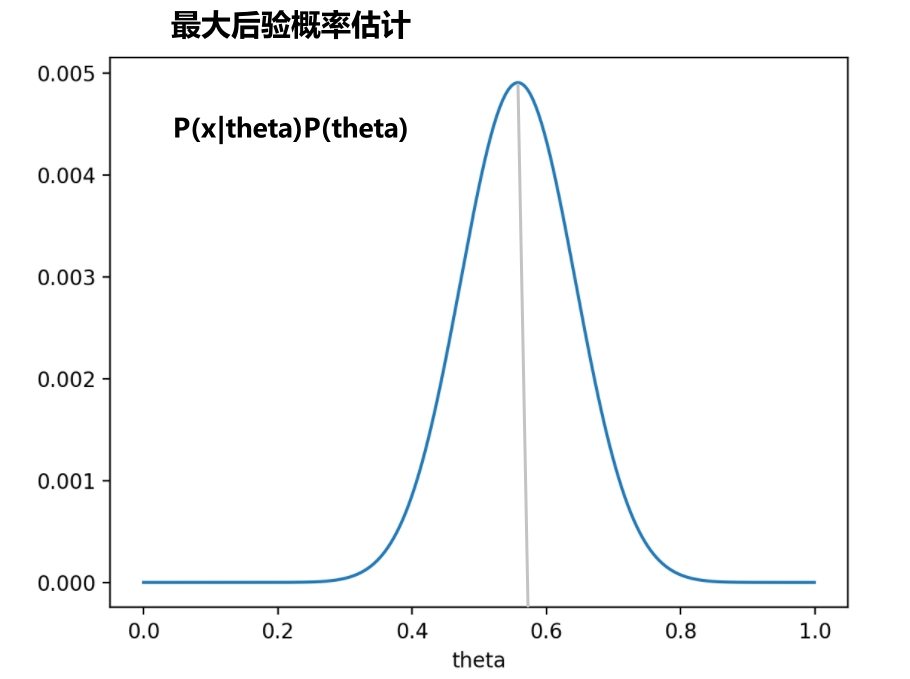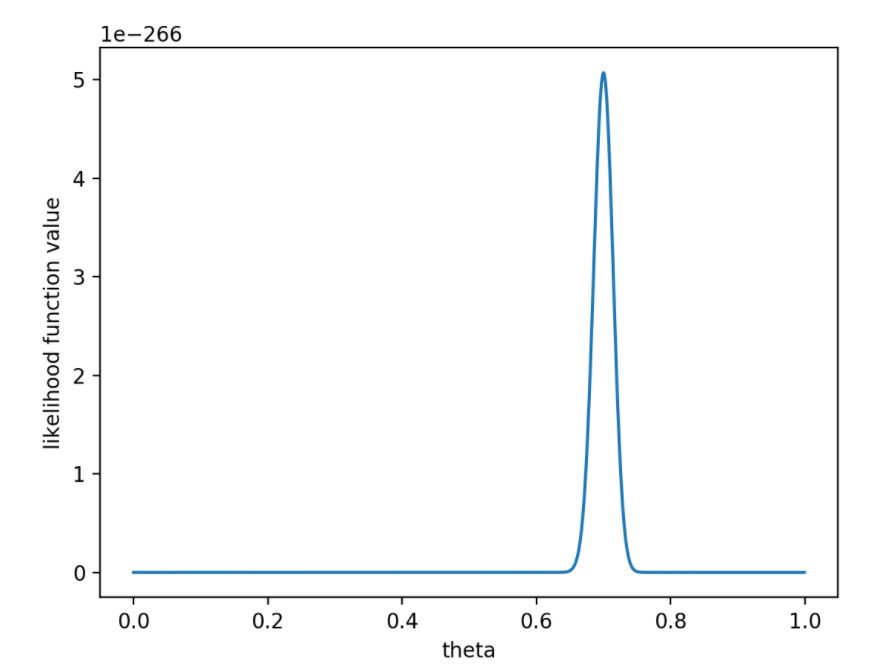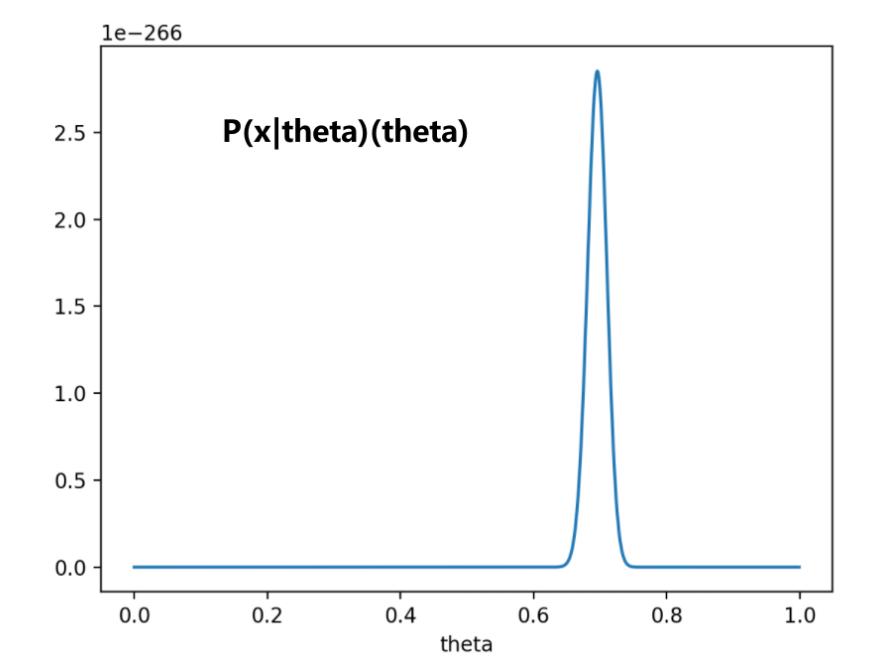$\underset{\theta}{\operatorname{argmax}} P(\theta \mid X)=\underset{\theta}{\operatorname{argmax}} \frac{P(X \mid \theta) P(\theta)}{P(X)} \propto \underset{\theta}{\operatorname{argmax}} P(X \mid \theta) P(\theta)=\underset{\theta}{\operatorname{argmax}} [\log P(X \mid \theta) + \log P(\theta)]$

# 五、 最大似然估计与最大后验估计的区别

## 频率学派和贝叶斯学派的争论

• 频率学派认为世界是确定的，有一个本体，这个本体的真值是不变的，即参数$\theta$是一个客观存在的固定值，我们的目标就是要找到这个真值或真值所在的范围。（世界上只有一个模型）

• 贝叶斯学派认为世界是不确定的，人们对世界先有一个预判，而后通过观测数据对这个预判做调整，我们的目标是要找到最优的描述这个世界的概率分布。。换言之，模型参数$\theta$是一个随机变量，服从一个概率分布（世界上有多个模型，每个模型出现的概率不同），我们首先根据主观的经验假定$\theta$的概率分布式$P(\theta)$（先验分布，例如高斯分布、指数分布，往往并不准确），然后根据观察到的新信息（数据集$X$）其进行修正。

## MLE和MAP的异同

• 极大似然估计MLE是求让$P(x|\theta)$最大的模型（它认为不同模型出现的概率相同，即$P(\theta)$是一个常数或者说它认为世界上只有一个模型）
• 最大后验概率估计MAP是求让$P(x|\theta)P(\theta)$最大的模型（它认为不同模型出现的概率不同）

• 高中数学有一个著名的概率问题，“一枚硬币连续投了五次都是正面，那么第六次投还是正面的概率是多少？”机智的高中生会想，这骗得了我？这是独立重复实验，概率还是0.5！（因为这个高中生有先验知识，根据常识硬币正面朝上的概率=0.5最常见
• 可如果一枚硬币连续投了一百次都是正面呢？一亿次都是正面呢？是否还要坚信出现正面的概率是0.5？这个硬币会不会被人动了手脚，就只有正面呢？（说明在具体实验中，先验知识可能并不准确）
• 当大量的事实摆在我们面前，随着数据越来越多，人应该越来越相信这个硬币有问题，越来越不相信硬币的概率是0.5，这才是最自然而然的感觉，这才是动态的看待问题，而不是机械僵化的看待问题，而这种直觉背后就是贝叶斯思想。（先验的影响越来越小

## 最大后验估计与L1 L2正则之间的关系

MAP的奥妙之处在于我们可以通过先验的方式给模型灌输一些信息，比如模型的参数$\theta$可能服从高斯分布，这样我们可以假定先验就是高斯分布

$P(\theta) = \frac{1}{\sqrt{2 \pi} \sigma} e^{-\frac{(\theta-\mu)^{2}}{2 \sigma^{2}}}$，其中$\mu$和$\sigma^2$是先确定好的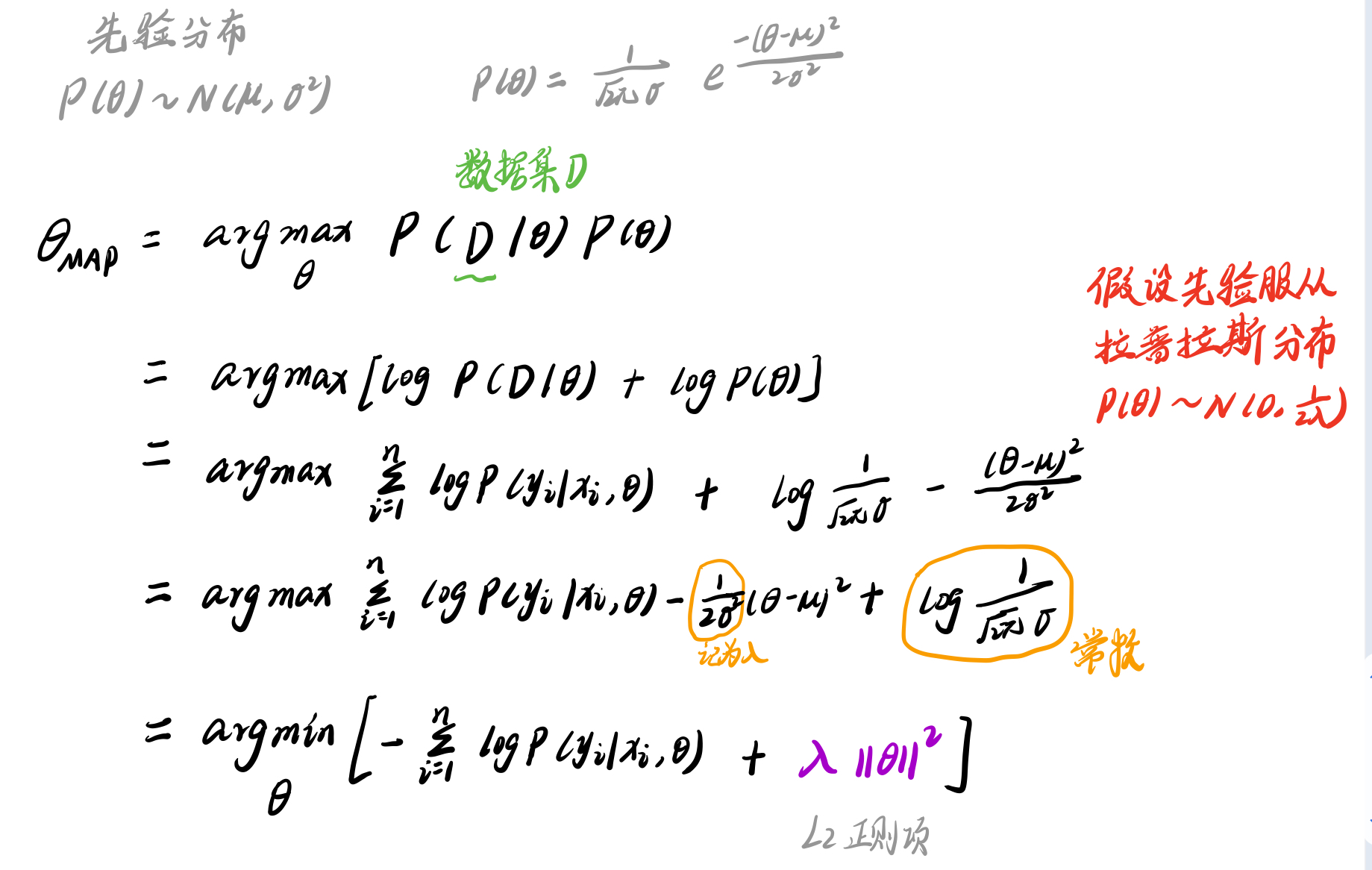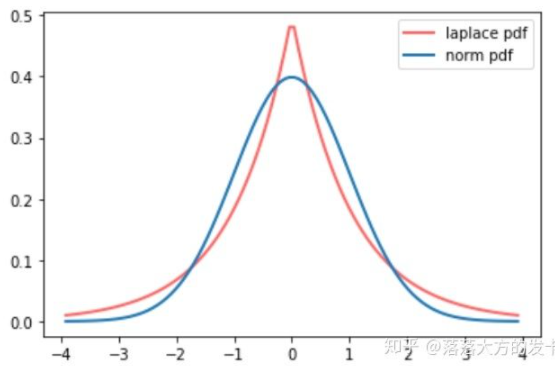$f(x \mid \mu, b)=\frac{1}{2 b} e^{-\frac{|x-\mu|}{b}}$，其中$\mu$和$b$是先确定好的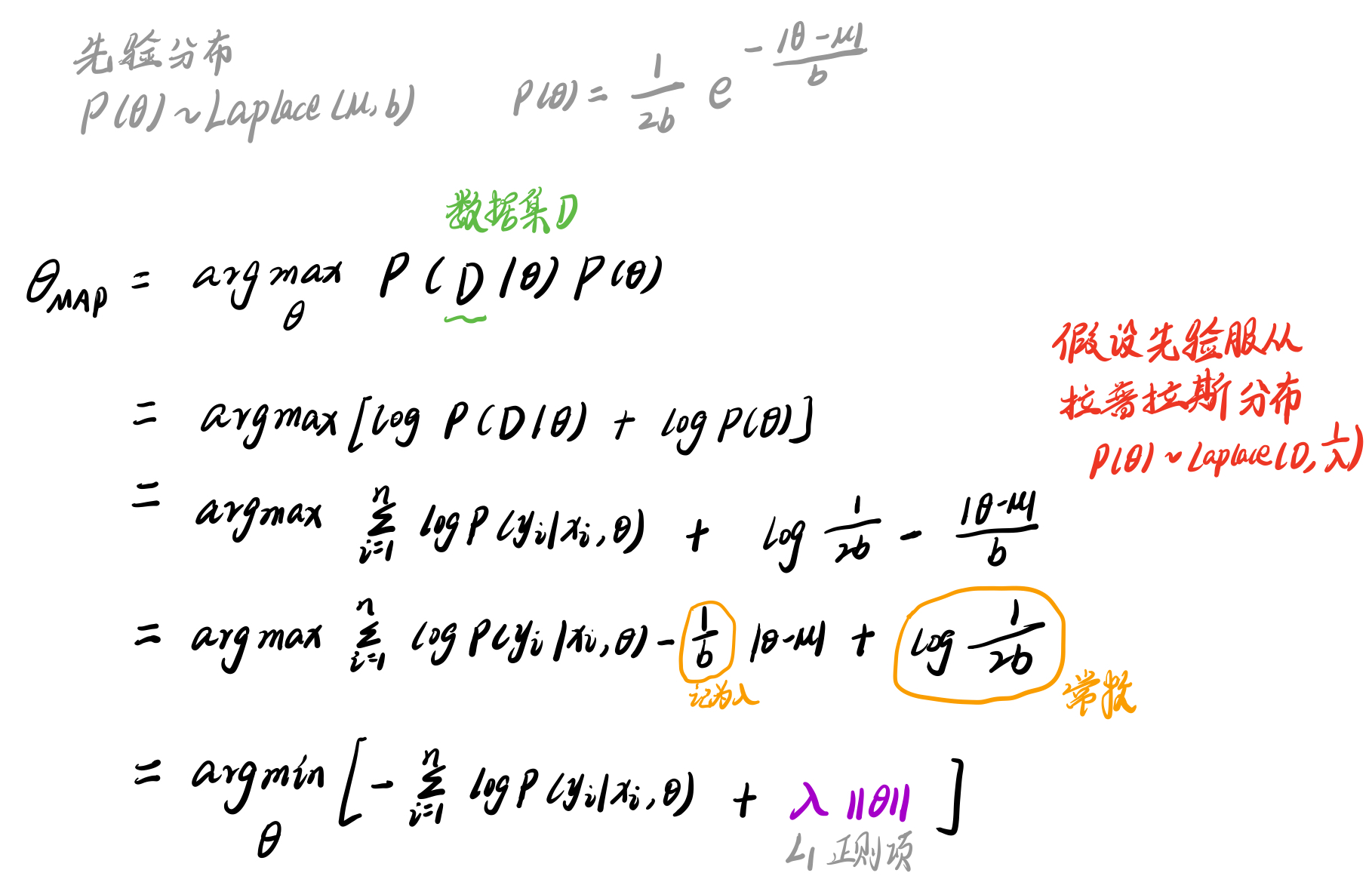• 假设参数的先验概率服从高斯分布，相当于原来损失函数加入了L2正则
• 假设参数的先验概率服从拉普拉斯分布，相当于原来损失函数加入了L1正则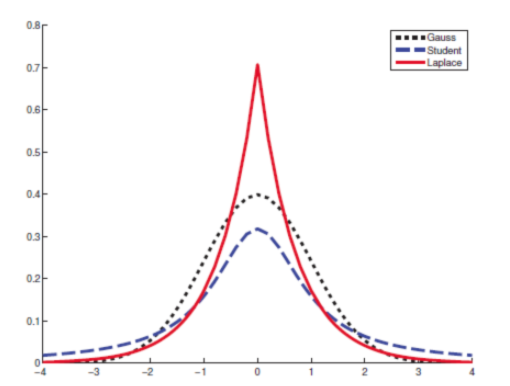# 六、进阶知识

## 贝叶斯公式理解（图解）$X$（已经确定）是某件已经发生的事情，$\theta$（变量）是某个原因。有了前面的知识铺垫，应该很容易明白为什么上面$P(X|\theta)$是似然函数而不是概率函数了吧

• X表示老板生气了
• $\theta$是表示某种原因
• 可能的原因1：员工迟到
• 可能的原因2：昨晚和老婆吵架了
• 可能的原因3：员工泄漏资料

$P(员工迟到 | 老板生气) =\frac{P(老板生气 | 员工迟到)P(员工迟到)}{P(老板生气)}= \frac{P(老板生气 | 员工迟到)P(员工迟到)}{P(老板生气 | 员工迟到)P(员工迟到) + P(老板生气 | 原因2)P(原因2) + P(老板生气 | 原因3)*P(原因3)}$

## 贝叶斯估计

Author:
Reprint policy: All articles in this blog are used except for special statements CC BY 4.0 reprint polocy. If reproduced, please indicate source SHWEI !

Previous【NIPS 2016图神经网络论文解读】Variational Graph Auto-Encoders (VGAE) 基于VAE的图变分自编码器

2021-12-10
Next【ICLR 2018图神经网络论文解读】Graph Attention Networks (GAT) 图注意力模型

2021-11-23
TOC